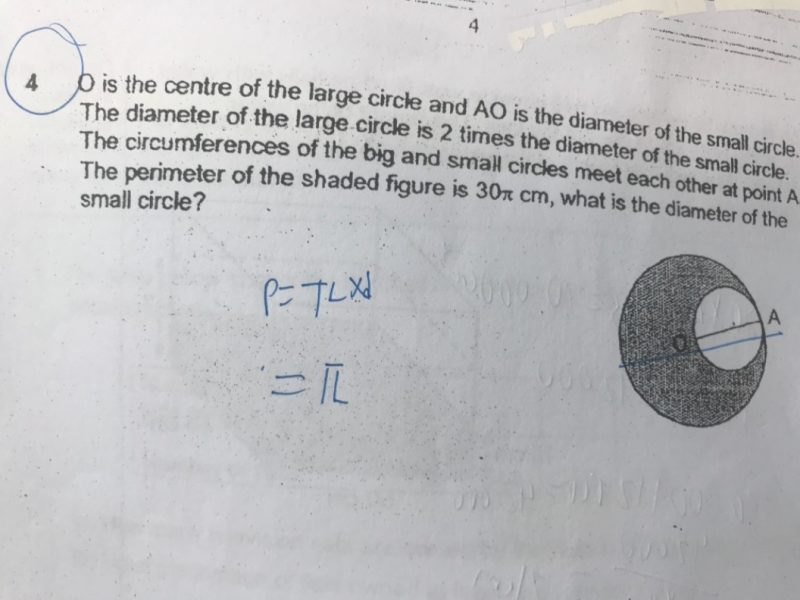# QuestionHi,

Can anyone help me to solve this question?

Thanks

If d is the diameter of the small circle, then diameter of big circle is 2d.

Perimeter of shaded figure = circumference of big circle + circumference of small circle

Circumference of big circle -> π(2d) = 2πd

Circumference of small circle -> πd

2πd + πd = 30π

3πd = 30π

d = 30π ÷ 3π = 10 cm

0 Replies 0 Likes

Ans: 10 cm

Let the diameter AO be d, and the diameter of the bigger circle be 2d.

Then, π(2d) + π(d) = 30π

3dπ = 30π

3d = 30

d = 10

0 Replies 0 Likes